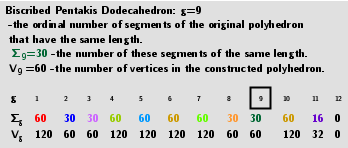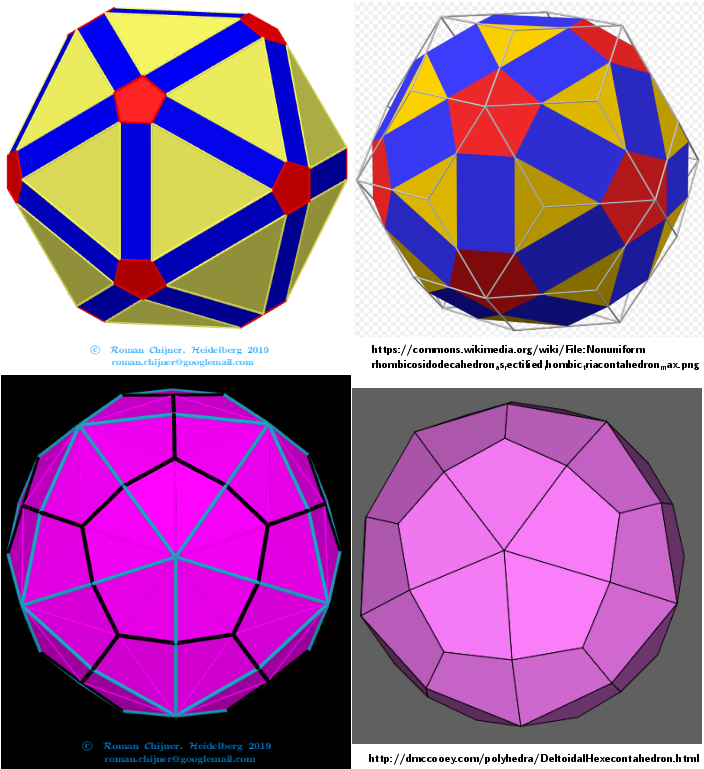# Rhombicosidodecahedron (V=60) from Biscribed Pentakis Dodecahedron for the case of trisection of its 9th-order segments(Variant2)

A polyhedron is constructed whose V=60 vertices are the points of the trisection of the segments the same length 9th-order(g=9) of the Biscribed Pentakis Dodecahedron. Geometric Constructions are in Applet: Series of polyhedra obtained by trisection (truncation) different segments of the original polyhedron, and the resulting polyhedra in Applet: Serie of polyhedra obtained by trisection (truncation) segments of the Biscribed Pentakis Dodecahedron.## 3. Properties of polyhedra

as Rhombicosidodecahedron
 Vertices: 60  (60) Faces: 62  (20 equilateral triangles + 30 squares + 12 regular pentagons) Edges: 120
Dual as Deltoidal Hexecontahedron Vertices: 62 (30 + 20 + 12) Faces: 120 (acute triangles) Edges: 180 (60 short + 60 medium + 60 long)Comparing my images and from sources: Rhombicosidodecahedron-Deltoidal hexecontahedron https://en.wikipedia.org/wiki/Rhombicosidodecahedron http://dmccooey.com/polyhedra/Rhombicosidodecahedron.html https://en.wikipedia.org/wiki/Deltoidal_hexecontahedron; http://dmccooey.com/polyhedra/DeltoidalHexecontahedron.html